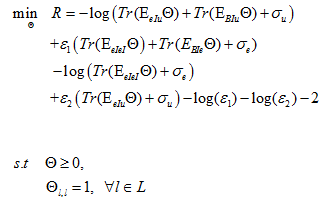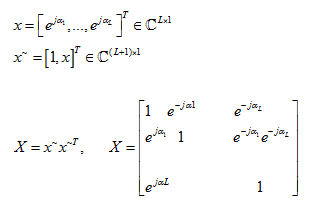# Why the diagonal matrix?

Hello
When I use cvx to optimize a problem, I also have a square matrix variable that is not diagonal according to the calculations on paper.
But the answer I get from MATLAB and cvx is diagonal.
Does any of you know where this problem could be coming from?
Thank you very much for your help.and my code is:
clc,close all,clear
ep1 =0.0086;
ep2 =0.0031;
var_u =10.^(-50/10);
var_e = var_u;
L=4;
E_BuII=46.6770;
G_eIuu=14.37;
E_BeII=65.76;
g_eIeI=8.6689;
n=L+1;
cvx_begin %sdp %quiet %cvx_precision best
variable X(n,n) hermitian %complex semidefinite hermitian
X == hermitian_semidefinite(L+1);
minimize -(log(trace(XG_eIuu)+var_u+trace(XE_BuII))/log(2))-(log(trace(Xg_eIeI)+var_e)/log(2))+ep1(trace(XG_eIuu)+var_u)-(log(ep1)/log(2))+ep2(trace(Xg_eIeI)+var_e+trace(XE_BeII))-(log(ep2)/log(2))-2;
subject to
for i=1:L+1
X(i,i)==1;
end
X == hermitian_semidefinite(L+1);
cvx_end
my results is:

Why do you think it should not be diagonal?

Because according to the formulas, I’m looking for a matrix as follows and that I want it not to be full-rankIt confuses me there are complex number in your objective, for example the trace of a complex matrix. And base on your description, you are doing semidefinite relaxation, which in most cases, doesn’t gurantee that the result be rank 1.

X is a matrix whose condition is that the original diameter is 1, so its trace is equal to a real value so it does not cause a problem with the objective function.

What I do ensures that my matrix is not full-ranking?

I don’t know if there is a way. In most cases you need to do gaussian randomization after you get the result matrix( if it is not rank 1), you might need to read the whole paper carefully.

Thank you very much for your help
Can you tell me how I should use Gaussian Randomization or do you have a sample code?

No, I don’t. Maybe you can trace the references in your paper.